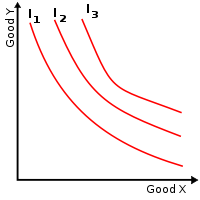# Ordinal utility

﻿
Ordinal utility

Ordinal utility theory states that while the utility of a particular good or service cannot be measured using a numerical scale bearing economic meaning in and of itself, pairs of alternative bundles (combinations) of goods can be ordered such that one is considered by an individual to be worse than, equal to, or better than the other. This contrasts with cardinal utility theory, which generally treats utility as something whose numerical value is meaningful in its own right.

## Indifference curve mappings

When a large number of bundles of goods are compared, the preferences of the individual can be seen. This information is usually put together on a graph called an indifference map. One of these is shown below:Each indifference curve is a set of points, each representing a combination of quantities of two goods or services, all of which combinations the consumer is equally satisfied with. The further a curve is from the origin, the greater is the level of utility. The slope of the curve (the negative of the marginal rate of substitution of X for Y) at any point shows the rate at which the individual is willing to trade off good X against good Y maintaining the same level of utility. The curve is convex to the origin as shown assuming the consumer has a diminishing marginal rate of substitution. It can be shown that consumer analysis with indifference curves (an ordinal approach) gives the same results as that based on cardinal utility theory — i.e., consumers will consume at the point where the marginal rate of substitution between any two goods equals the ratio of the prices of those goods (the equi-marginal principle).

## Revealed preference

Revealed preference theory addresses the problem of how to observe ordinal preference relations in the real world. The challenge of revealed preference theory lies in part in determining what goods bundles were foregone, on the basis of the being less liked, when individuals are observed choosing particular bundles of goods. 

## Ordinal utility functions

An ordinal utility function describing a consumer's preferences over, say, two goods can be written as

u(x,y)

where x and y are the quantities of the goods consumed. Both partial derivatives of this function are positive if the consumer prefers more of both goods. But the same preferences could be expressed as another utility function that is a monotonic transformation of u:$g(x, y) \equiv f(u(x, y)),$

where f is any globally increasing function. Utility functions g and u give rise to identical indifference curve mappings. Thus in ordinal utility theory, there is no concept of diminishing marginal utility, which would correspond to the second derivative of utility being negative. For example, even if u has a negative second derivative with respect to x, the equivalent utility function g may have a positive second derivative with respect to x.

Wikimedia Foundation. 2010.

### Look at other dictionaries:

• ordinal utility — (Economics) utility that can be measured quantitatively (and not numerically) …   English contemporary dictionary

• utility and value — ▪ economics Introduction       in economics, the determination of the prices of goods and services.       The modern industrial economy is characterized by a high degree of interdependence of its parts. The supplier of components or raw materials …   Universalium

• Cardinal utility — In economics, cardinal utility is a theory of utility under which the utility (roughly, satisfaction) gained from a particular good or service can be measured and that the magnitude of the measurement is meaningful. Under cardinal utility theory …   Wikipedia

• Marginal utility — In economics, the marginal utility of a good or service is the utility gained (or lost) from an increase (or decrease) in the consumption of that good or service. Economists sometimes speak of a law of diminishing marginal utility, meaning that… …   Wikipedia

• Expected utility hypothesis — In economics, game theory, and decision theory the expected utility hypothesis is a theory of utility in which betting preferences of people with regard to uncertain outcomes (gambles) are represented by a function of the payouts (whether in… …   Wikipedia

• Quasilinear utility — In economics and consumer theory, quasilinear utility functions are linear in one argument, generally the numeraire. Formally, for example, such a utility function could be written U(x,y) = u(x) + by, where b is a positive constant. Then if u… …   Wikipedia

• Полезность — UTILITY 1. Удовлетворение или удовольствие, которое получает человек от потребления товара или услуги. Полезность категория субъективная: то, что полезно для одного человека, может быть абсолютно бесполезно для другого. По этой причине полезность …   Словарь-справочник по экономике

• Функция полезности — UTILITY FUNCTION Зависимость, отражающая количество товаров и услуг, потребляемых человеком: где Ut совокупная полезность, х1, х2 ... хn потребляемые продукты. Чтобы  достичь  потребительского  равновесия  (см.  Consumer equilibrium), человек… …   Словарь-справочник по экономике

• Ординалистская (порядковая) полезность — ORDINAL UTILITY Субъективная полезность или удовлетворение, которое извлекается от потребления продукта, измеренное по принципу относительности. В концепции ординалистской полезности признается, что невозможно точно измерить величину полезности в …   Словарь-справочник по экономике